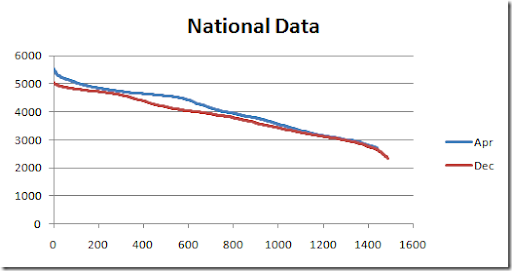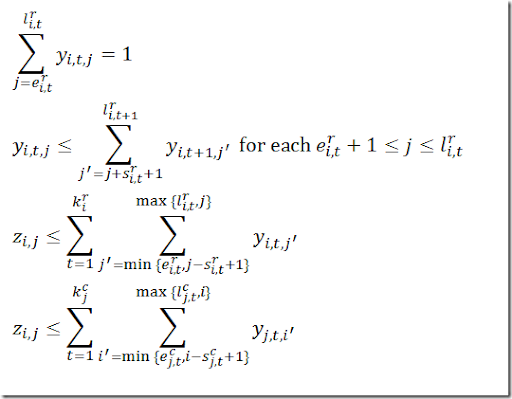## Wednesday, March 31, 2010

### Creating Load Duration Curves with GAMS

In power generation analysts often want to see a Load Duration Curve (http://en.wikipedia.org/wiki/Load_duration_curve). This involves sorting, a task that is not always immediately obvious how to do in GAMS. Here is some optimized code to perform the creation of a load curve:

alias (st,month,m);
alias (d,day);
set k /k1*k10000/;

dt(d,tp)\$

* map (d,tp) onto a 1 dim set k.
option map(dt:k);

set monthsub(month) 'only months that are really used';
monthsub(m)\$(

* gdxrank only sorts one dimensional parameters so we use mapping
parameter unsorted(k),sorted(k);
parameter indx(k);
\$libinclude rank
loop(monthsub(m),
unsorted(k) =
\$  libinclude rank unsorted k indx
sorted(k + (
card(k) + 1 - indx(k) - ord(k)) ) = unsorted(k);
sum(map(dt,k),sorted(k));
);

It uses some obscure GAMS syntax. The advantage is that they are fast but obviously this code is difficult to read: it is no longer intuitive what is happening here. The resulting graphs in the GAMS IDE and in Excel are:## Wednesday, March 24, 2010

### GAMS/Baron documentation

“Variable Priorities. BARON implements branch-and-bound algorithms involving convex relaxations of the original problem. Branching takes place not only on discrete variables, but also on continuous ones that are nonlinear. Users can specify branching priorities for both discrete and continuous variables.”

Unfortunately GAMS does not allow you to set priorities on continuous variables:

 1  variable x;    2  x.prior = 2; ****        \$323 **** 323  Priorities can only be used on discrete variables

I believe the storage is shared with x.scale, but I am not sure if we can trick Baron by using a .scale suffix.

As said in the comment, the priorities are specified in the option file instead of the GAMS language. That is a reasonable alternative although we have slightly less expressiveness.

## Monday, March 22, 2010

### MINLP/Baron

This looks really neat, but not being a mathematical programming consultant, I am a bit mystified by references to MINLP and BARON. I assume that the former is an algorithm and the latter is mathematical software. Can it be reimplemented in R?

Sorry about that (I use these terms daily and too often assume they are well-known in the "normal" world):

MINLP is Mixed Integer Nonlinear Programming, i.e. a constrained nonlinear optimization problem where some of the variables can assume only discrete values.

Here is an overview: http://coral.ie.lehigh.edu/presentations/siopt-05-minlp-handout.pdf

Baron is actually a very complex algorithm. I doubt it is easy to re-implement this in R, but an R interface may be doable. Here is some information: http://archimedes.cheme.cmu.edu/baron/baron.html

### Looks difficult to me (3)

Actually in posting http://permalink.gmane.org/gmane.comp.lang.r.general/183891 there is a little example in R. The graph looks like:

The MINLP/Baron solution for this data set is a little bit better in terms of total area:I am surprised how difficult this is to predict just by looking at the picture. Note that implementing the suggested side constraints would be trivial. Initially I thought it may even improve the performance but this is not the case: the problem becomes a little bit harder. For the 100 point problem above I added the constraint

0.5 ≤ a/b ≤ 2

It is better to multiply by b to get rid of the division, so actually I added:

0.5b ≤ a ≤ 2b

This gives:

The rectangle is shifted a little bit to achieve a slightly larger area. Of course adding upper bounds of 0.5 to a and b will speed up things a lot.

### Looks difficult to me (2)

I tried a simple minded MINLP formulation, and solved this with Baron:

This actually worked! Even for n=100 and n=200. Note that it is important to use a global solver as there are many inferior local solutions. As I solved with OPTCR=0 these are really proven global optimal solutions. I assumed the rectangle can not be rotated and must be aligned with the x and y axes. Here are some results.

Here are the corresponding plots of the solutions:Of course faster special purpose algorithms exist for this problem.

## Sunday, March 21, 2010

### Painting by Numbers (2)

As a follow up on Painting by numbers here is a big one:There is also one I cannot solve quickly (may be an error in reproducing the input on my side):To make sure we are hitting the limits of MIP solver technology here and this is not due to some transcription error in the input data I should try this data with some other solver, e.g. a constraint programming system. (I did a simple check on the input: the count of the cells covered by horizontal clusters must be the same as the count of the cells covered by the vertical clusters).

### Looks difficult to me

`For an application in image processing -- using R for statistical purposes -- Ineed to solve the following task:Given n (e.g. n = 100 or 200) points in the unit square, more or less randomlydistributed. Find a rectangle of maximal area within the square that does notcontain any of these points in its interior.If a, b are height and width of the rectangel, other constraints may have to beimposed such as  a, b <= 0.5  and/or  0.5 <= a/b <= 2.0 . The rectangle isallowed to touch the border of the square.For each new image the points will be identified by the application, like allstars of a certain brightness on an astronomical picture. So the task will haveto be performed several times.I assume this problem is computationally hard. I would like to find a solutionthat is reasonably fast for  n = 100..200  points. Exhaustive search along thex, y coordinates of the points will not be fast enough.`

`I doubt there is easy way to solve this. At least it is not obvious to me.`

`PS: see also http://yetanothermathprogrammingconsultant.blogspot.com/2010/03/looks-difficult-to-me-2.html`

## Sunday, March 14, 2010

### Painting by numbers

A client of mine was trying to implement as an exercise the models in Robert A. Bosch, "Painting By Numbers", OPTIMA 65, May 2001. He was using Excel/Frontline. (Being snowed-in for some days causes people to do strange things).  My guess is that this is not completely trivial. The indexing is somewhat complicated, as illustrated by some of the messy math:However it is not too difficult to use both Excel as front-end and a real modeling system to deal with the model:

It looks like this picture is slightly different than in the Bosch article (figure 4).

Basically we export the raw data immediately (i.e. with minimal preprocessing) to the GAMS model and ship the final solution (which cells to paint black) back to Excel. IMHO, this is often more convenient than trying to do everything in Excel.

Turns out the well-known mip solvers such as Gurobi and Cplex do a good job on (most of) these models. In http://webpbn.com/survey/ the performance looks dismal for this formulation (see last column labeled Bosch/glpk) but I see much better results. First this formulation can easily handle empty rows, so the ‘X’ entries can disappear. Second for most models zero nodes are needed, so many models solve fairly quickly. That should remove some of the entries with ‘-‘.

## Sunday, March 7, 2010

### Expensive graph

For a report on a model I produced some summary graphs that turned out to be rather time-consuming to generate.

Each point represents the solution of a different MIP model. In total we have 9 series × 21 points = 189 models. Each model takes between 10 seconds and 500 seconds to solve, on average about 200 seconds. So this single chart took 10 hours to generate. And there were a few others like this.

## Friday, March 5, 2010

### Calculating the IRR (Internal Rate of Return)

In a GAMS model where I try to select good investment projects (and find an optimal schedule when to implement them) at the end we wanted to show the IRR (Internal Rate of Return). There is no good closed formula for this, so my first approach to solve a series of one-variable, one-equation nonlinear models:

parameter cf(y) 'cashflows';
equation ecashflow;
variable irr;
irr.LO=0;
irr.UP=100;

ecashflow..
sum(y,cf(y)/[(1+irr)**(ord(y)-1)]) =e= 0;

model mirr /ecashflow/;

This can then be solved as follows:

loop(p\$(cashflow(p,'cashflow','sum')>0),
cf(y) = cashflow(p,
'cashflow',y);
irr.L = 0.01;

solve mirr using cns;
cashflow(p,
'irr','sum') = irr.L;
);

The overhead can be reduced somewhat by solving as a single larger model:

parameter cf(p,y);
equation ecashflow(p);
variable irr(p);
irr.LO(p)= 0;
irr.UP(p)= 100;

set psub(p) 'subset';

ecashflow(psub)..

sum(y,cf(psub,y)/[(1+irr(psub))**(ord(y)-1)]) =e= 0;

model mirr /ecashflow/;

psub(p)\$(cashflow(p,
'cashflow','sum')>0) = yes;
cf(psub,y) = cashflow(psub,
'cashflow',y);
irr.L(psub) = 0.01;
solve mirr using cns;
cashflow(psub,
'irr','sum') = irr.L(psub);

Actually this was a little bit more stable than using the Excel function =IRR(). For the Excel function I needed different starting points to achieve convergence.

The last formulation is actually a square system of nonlinear equations, with only Jacobian entries on the diagonal. I.e. a solver like CONOPT can solve this completely in the presolver. In essence it will do internally exactly what I wrote in the first version: solving a series of 1 variable, 1 equation problems.

As the number of projects is relatively small for this model (<100), this nonlinear model fits in the demo size limits. So I don’t have to charge the client for an extra solver (the main model is a reasonably sized MIP).